# Circuit Analysis-I January 2013

UNIT – I

1. (a) A 50 |i/-‘ capacitor, when connected in series with a coil having 40 Q resistance, resonates at 1000 Hz. Find the inductance of the coil. Also obtain the circuit current if the applied voltage is 100 V. Also calculate the voltage across capacitor and the coil at resonance.

(b) For the resistive network shown below, draw the graph and tree of the network. Also develop the fundamental cut-set matrix.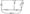OR

(a)  Draw the dual of the following network.(b) Show the cut-sets for the graph of the network shown below and develop the fundamental cut-set matrix.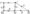UNIT – II

2. (a)  State and explain compensation theorem.

(b) Draw Thevenin’s equivalent circuit to the Right of terminal ‘ab’ in circuit shown below.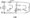(a)  Derive the condition for maximum power transfer and explain maximum power transfer theorem.

(b) Find current I in the circuit shown below using superposition theorem.

UNIT – III

3. (a)With the help of phasor diagram draw the relationship between line voltage and phase voltage, line current and phase current for balanced 3-phase delta connected load.

(b) A load of (3+ /4) ohms is connected in each phase of a delta connected network. If a balanced 3-phase 100 volt supply is applied between lines, calculate line current and phase current.

OR

(a) What do you mean by power triangle ? Explain active, reactive and apparent power with example.

(b) In the circuit shown below find the following (i) current

(ii)  voltage across each element (iii) power factor of the circuit

(iv)  power consumed.

UNIT – IV

4.(a)Obtain Fourier series of the given waveform.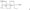(b) Derive the formula for average value and effective value for half wave rectified waveform. Also calculate form factor for this waveform.

OR

(a) Find the Fourier expansion of the given waveform.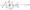(b) Explain the different kinds of symmetry in non-sinusoidal waves.

UNIT – V

5. (a) In the figure shown below steady state is reached. At t = 0, switch k is suddenly opened. Find the current through inductor after t = 1 sec. 2.0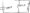(b) Find expression for step response of R-L series circuit.

OR

(a)  Obtain the Fourier series expansion in trigonometric form of full wave rectified waveform of amplitude A.

(b) In the figure shown below switch k is closed at position A at t = 0. After the lapse of 10~3 sec, the switch is moved to position B. Determine the expression for complete current.### 4 thoughts on “RTU Previous Question Papers BE EE 3rd Semester Circuit Analysis-I January 2013”

1.I want to realfact with ca

•can I get the answer of this paper

2.3.